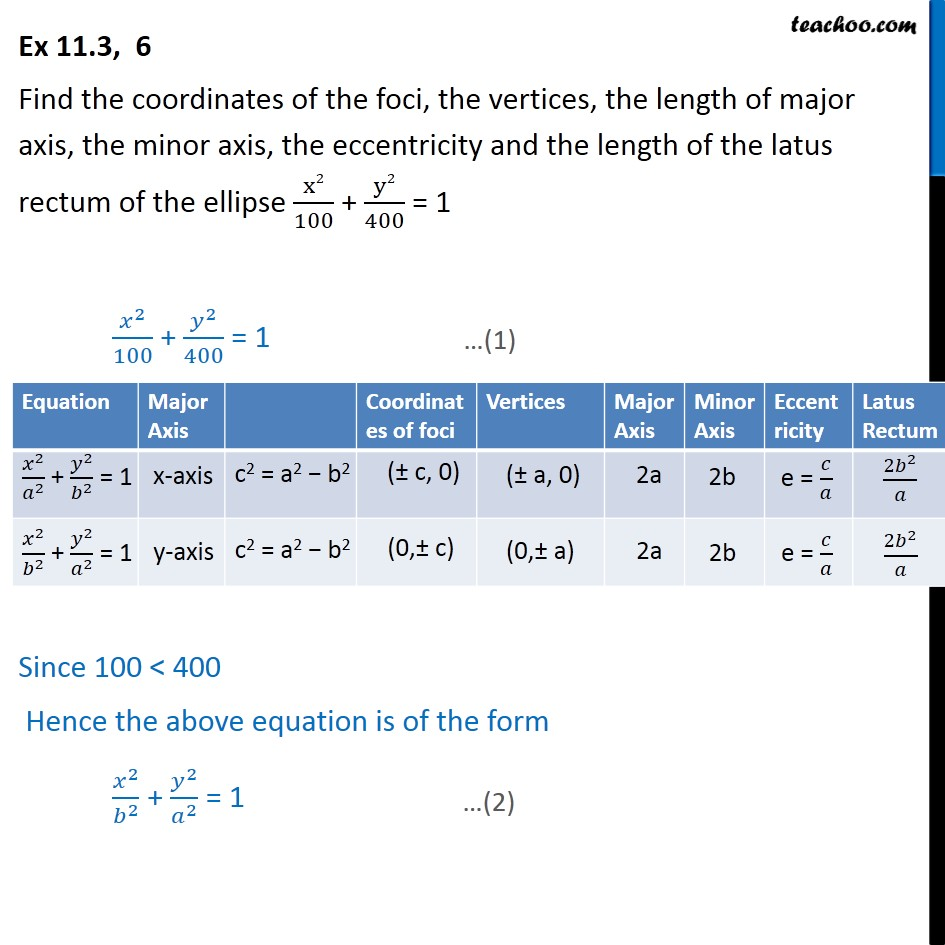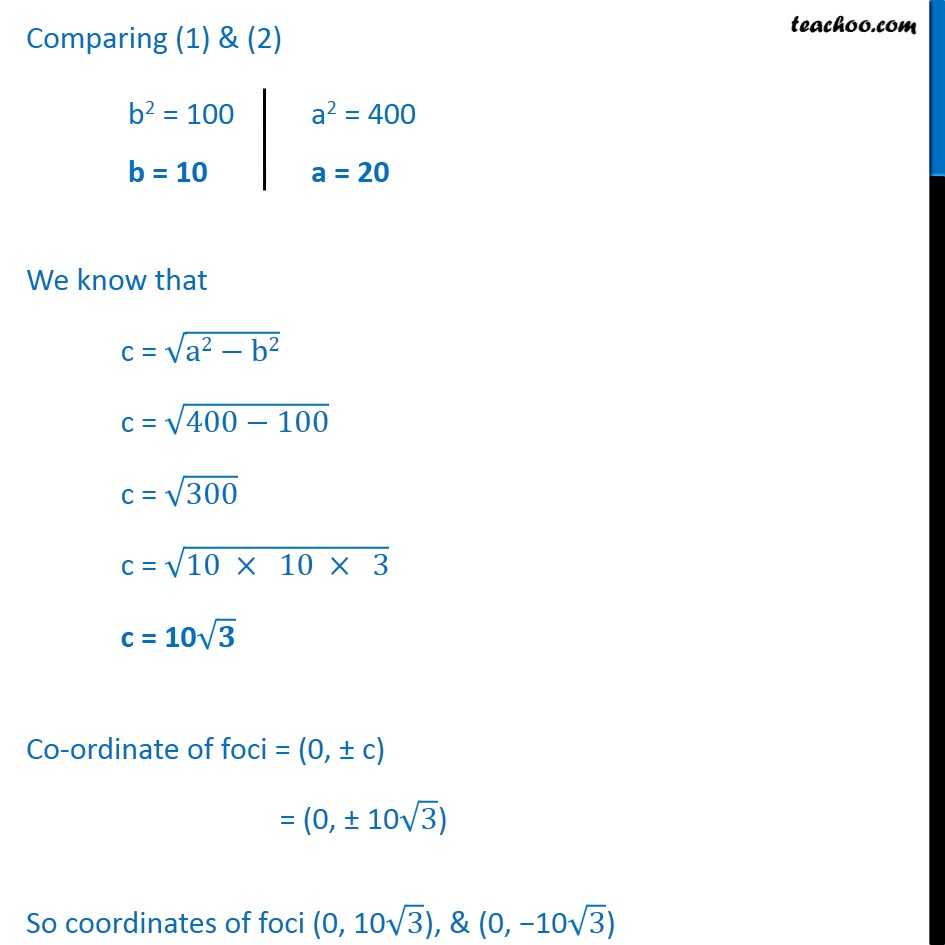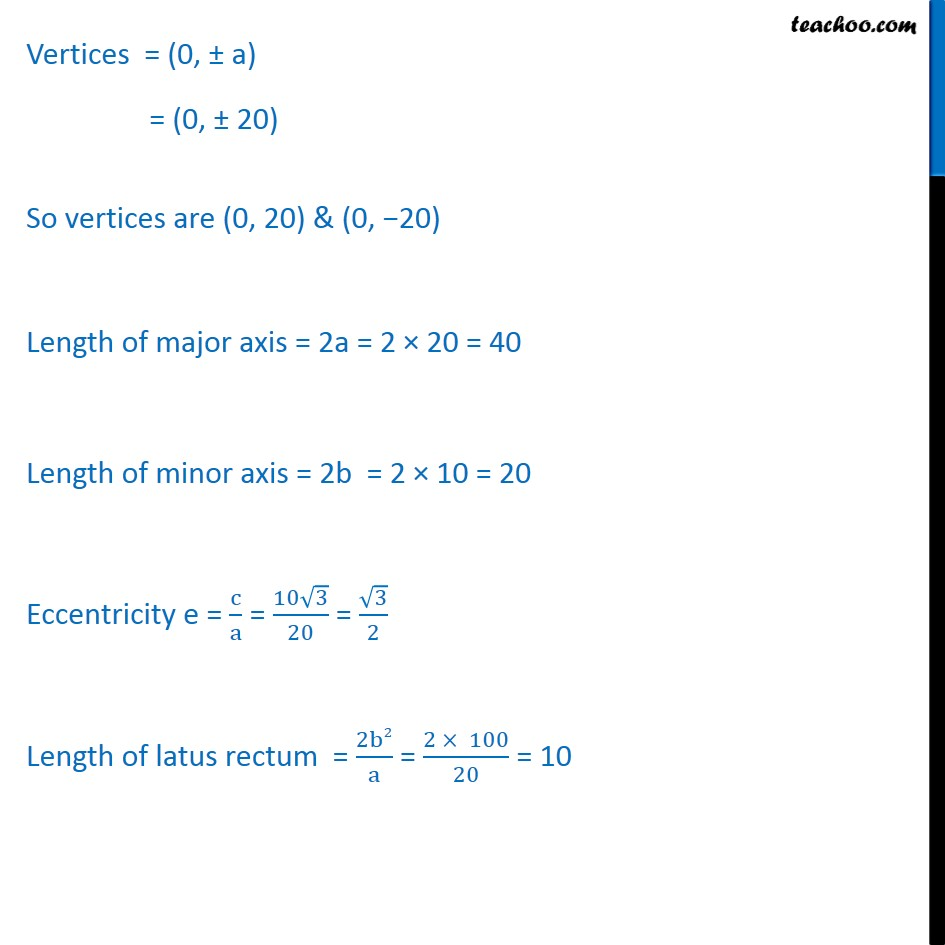1. Chapter 11 Class 11 Conic Sections
2. Serial order wise
3. Ex 11.3

Transcript

Ex 11.3, 6 Find the coordinates of the foci, the vertices, the length of major axis, the minor axis, the eccentricity and the length of the latus rectum of the ellipse ﷐x2﷮100﷯ + ﷐y2﷮400﷯ = 1 ﷐﷐𝑥﷮2﷯﷮100﷯ + ﷐﷐𝑦﷮2﷯﷮400﷯ = 1 Since 100 < 400 Hence the above equation is of the form ﷐﷐𝑥﷮2﷯﷮﷐𝑏﷮2﷯﷯ + ﷐﷐𝑦﷮2﷯﷮﷐𝑎﷮2﷯﷯ = 1 Comparing (1) & (2) We know that c = ﷐﷮a2−b2﷯ c = ﷐﷮400−100﷯ c = ﷐﷮300﷯ c = ﷐﷮10 × 10 × 3﷯ c = 10﷐﷮𝟑﷯ Co-ordinate of foci = (0, ± c) = (0, ± 10﷐﷮3﷯) So coordinates of foci (0, 10﷐﷮3﷯), & (0, −10﷐﷮3﷯) Vertices = (0, ± a) = (0, ± 20) So vertices are (0, 20) & (0, −20) Length of major axis = 2a = 2 × 20 = 40 Length of minor axis = 2b = 2 × 10 = 20 Eccentricity e = ﷐c﷮a﷯ = ﷐10﷐﷮3﷯﷮20﷯ = ﷐﷐﷮3﷯﷮2﷯ Length of latus rectum = ﷐2b2﷮a﷯ = ﷐2 × 100﷮20﷯ = 10

Ex 11.3

About the AuthorDavneet Singh
Davneet Singh is a graduate from Indian Institute of Technology, Kanpur. He has been teaching from the past 9 years. He provides courses for Maths and Science at Teachoo.